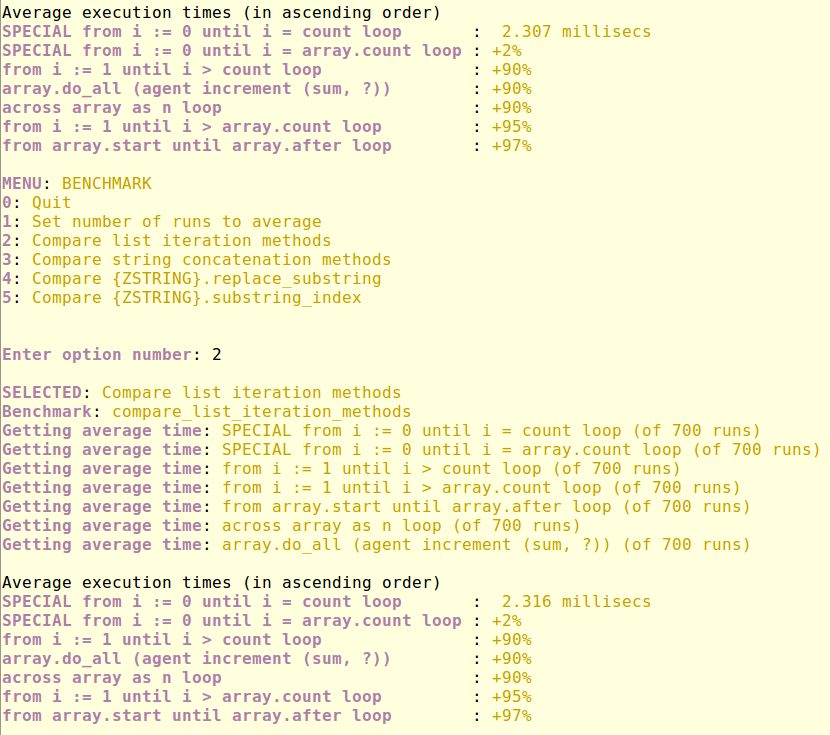# Benchmarking Five List Iteration Methods

by Finnian Reilly (modified: 2018 May 19)

## Introduction

The most commonly used list is type `ARRAYED_LIST`. When optimising your Eiffel code it is useful understand the performance implications of using different methods to iterate over a list. This article reports on the benchmarks for five types of iteration method, and also measures the difference that caching the array count as a local variable makes.

## Results Screenshot

The screenshot shows two tryies of the iteration comparison from the menu shell. In relative terms the results are identical. The benchmarking class EL_BENCHMARK_ROUTINE_TABLE displays the fastest time in millisecs. The remaining results are presented in ascending order of execution time relative to the first result.## Runtime Conditions

EiffelStudio version: 16 (16.05.9.8969 GPL Edition - linux-x86-64)

C Compiler: gcc version 4.8.4

Exe type: finalized exe

Assertions: off

Code inlining: level 2

OS: Linux Mint 17.1

CPU: Intel Core i7-3615QM CPU @ 2.30GHz

## Benchmark Conditions

The task of the loop is to add all the numbers from one to a million. Each routine benchmark is the average of 700 loop runs (`apply_count`) as expressed in this benchmarking routines. (Note a full garbage collect is carried out after each benchmark).

average_execution (action: ROUTINE; apply_count: INTEGER): DOUBLE local timer: EL_EXECUTION_TIMER; i: INTEGER do create timer.make timer.start from i := 1 until i > apply_count loop action.apply Memory.full_collect i := i + 1 end timer.stop Result := timer.elapsed_time.fine_seconds_count / apply_count end

## Benchmark Code

The code for these benchmarks can be inspected in the following classes. These classes are designed to make it easy to do quick performance comparisons for different styles of Eiffel code.

## Iteration Routines

These are the benchmarks in order of efficiency (fastest first)

### 1st. Cached SPECIAL iteration (2.3 ms)

Not surprisingly the fastest way to iterate over an arrayed list is to iterate over the inner `area` array of type `SPECIAL [INTEGER]` while caching the array count for the `until` condition with a local variable (`local_count = True`). The average time to execute is 2.3 millisecs. It is nearly twice as fast as all the other iteration types.

iterate_special_from_i_until_i_eq_count (array: SPECIAL [INTEGER]; local_count: BOOLEAN; sum: INTEGER_REF) local i, count: INTEGER do sum.set_item (0) if local_count then count := array.count from i := 0 until i = count loop sum.set_item (sum.item + array [i]) i := i + 1 end else from i := 0 until i = array.count loop sum.set_item (sum.item + array [i]) i := i + 1 end end end

### 2nd. SPECIAL iteration (+2%)

This benchmark uses the same method as in the 1st but does not cache the array count. This adds 2% to the execution time.

### 3rd. do_all & across & i_th (+90%)

The following 3 iteration methods tie for 3rd place.

iterate_do_all (array: ARRAYED_LIST [INTEGER]; sum: INTEGER_REF) do sum.set_item (0) array.do_all (agent increment (sum, ?)) end iterate_across_array_as_n (array: ARRAYED_LIST [INTEGER]; sum: INTEGER_REF) do sum.set_item (0) across array as n loop sum.set_item (sum.item + n.item) end end

Iterating using the `{ARRAYED_LIST}.i_th` function if you cache the array count to a local variable (`local_count = True`).

iterate_from_i_until_i_gt_count (array: ARRAYED_LIST [INTEGER]; local_count: BOOLEAN; sum: INTEGER_REF) local i, count: INTEGER do sum.set_item (0) if local_count then count := array.count from i := 1 until i > count loop sum.set_item (sum.item + array [i]) i := i + 1 end else from i := 1 until i > array.count loop sum.set_item (sum.item + array [i]) i := i + 1 end end end

### 4th. Uncached 'i_th' (+95%)

This uses routine `iterate_from_i_until_i_gt_count` with `local_count = False`. This mean the `until` condition has to call `array.count` on each iteration.

### 5th. start until after (+97%)

In last position is the start until after loop which is consistently slower than the other methods.

iterate_start_after_forth (array: ARRAYED_LIST [INTEGER]; sum: INTEGER_REF) do sum.set_item (0) from array.start until array.after loop sum.set_item (sum.item + array.item) array.forth end end